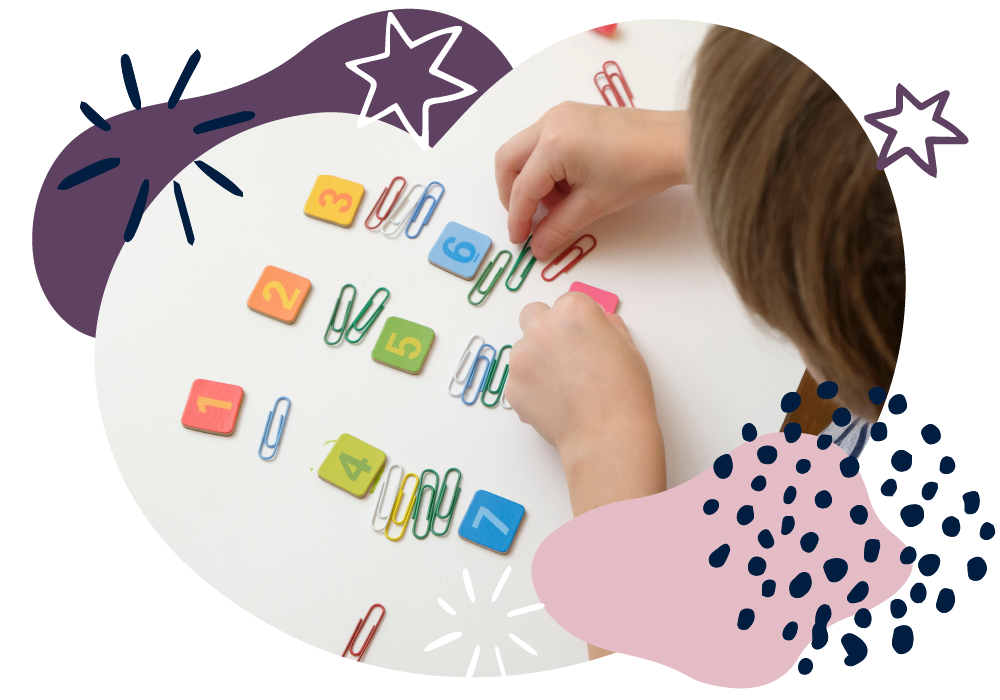We use cookies to enhance your experience on our website. By continuing to use our website, you are agreeing to our use of cookies. You can change your cookie settings at any time. Find out more# Maths: Age 7–8 (Year 3)

In Year 3, your child will continue to develop their understanding of numbers and start to calculate using formal written methods. They will learn a lot more about fractions, including tenths. They will find perimeters of 2D shapes, use the 24-hour clock, recognise angles, and start to use bar charts.

They’ll count in steps of 4, 8, 50 and 100 and order numbers to 1000. They’ll partition numbers into 100s, 10s and 1s, add and subtract three-digit numbers, and multiply two-digit by one-digit numbers. They will be taught the 3, 4, and 8 times tables and begin to add and subtract fractions.

Don’t worry if some methods that your child learns are new to you! There are still simple things you can do at home to support their development.

## How to help at home

You don’t need to be an expert to support your child with maths or help them develop a good sense of number! Here are three simple, but effective learning ideas that you can try with your child using everyday items at home.

### 1. Make arrays

Arrays  are shapes or objects arranged in a rectangle, such as a muffin tray or an egg box. Give your child some counters, buttons or beads, say 12. How many different arrays can they make? What calculations does each array show?

### 2. Play with food

Use foods with a regular shape (cake, pizza, cucumber slices) and ask questions like: Let’s cut this into 8 pieces, what fraction is each piece?

Find fractions of amounts using foods such as fishfingers or biscuits: There are 12 in the packet and 4 of us. What fraction can we each have? How many each is that?

### 3. Hunt for treasure

Hide some ‘treasure’ in a room and blindfold your child. Give them directions to find the treasure, such as: Turn two right angles clockwise, now take three steps forward. Use whole, half, quarter and three-quarter turns clockwise and anti-clockwise.

You’ll find more activity ideas in our Kids’ activities section

## What your child will learn at school

#### Number & place value in Year 3 (age 7–8)

In Year 3, children will be expected to recognise hundreds, tens and ones in 3-digit numbers (for example, 423 has 4 hundreds, 2 tens and 3 ones). This includes:

• counting in steps of 4, 8, 50 and 100
• reading, writing, comparing and ordering numbers to 1000
• finding 10 or 100 more or less than a number.

#### Addition & subtraction in Year 3 (age 7–8)

In Year 3, children will be expected to be able to use a range of strategies to solve problems mentally and learn formal written methods for column addition and column subtraction. This includes:

• adding and subtracting numbers with up to three digits
• estimating answers to problems before working them out accurately and checking using the inverse operation, i.e. using addition to check subtraction and vice versa
• explaining how they have solved a problem and why they chose a particular method.

#### Multiplication & division in Year 3 (age 7–8)

In Year 3, children will be expected to use a range of strategies to solve problems mentally and begin to learn formal written methods for short multiplication and short division. This includes:

• knowing and using multiplication and division facts for the 3, 4 and 8 times tables
• multiplying two-digit by one-digit numbers
• understanding that multiplication and division have an inverse relationship, i.e. they undo each other, and using this to check their calculations.

#### Fractions in Year 3 (age 7–8)

In Year 3, children will be expected to be able to compare and order fractions and begin to add and subtract fractions with the same denominator. This includes:

• counting up and down in tenths and connecting tenths to dividing by 10
• using unit and non-unit fractions
• understanding equivalent fractions, that is fractions that have the same value such as  .

#### Geometry in Year 3 (age 7–8)

In Year 3, children will be expected to use accurate mathematical language to describe properties of a wider range of symmetrical and non-symmetrical shapes. This includes:

• drawing 2D shapes and making 3D shapes
• identifying angles greater or less than a right angle
• identifying horizontal, vertical, parallel and perpendicular lines.

#### Measurement in Year 3 (age 7–8)

In Year 3, children will be expected to be able to add and subtract measurements, tell the time to the nearest minute and compare durations of time. This includes:

• measuring and adding to find the perimeter of 2D shapes
• telling the time using 12-hour and 24-hour clocks, including Roman numerals I to XII for 1 to 12
• adding and subtracting amounts of money to give change.